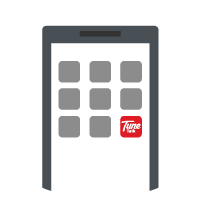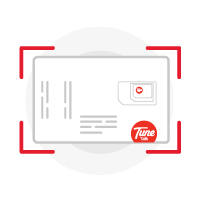FREE 36GB Data up to 12 months* when you join Tune Talk today!

All you have to do is to get a Tune Talk VALUE Prepaid Pack and make a minimum top up of RM30 within 7 days upon activation.

Promotion valid for limited time only.

TYPE of registration  FREE DATA Month(s) TOTAL DATA
New Tune Talker
(New number)
3GB x6 Months 18GB
New Tune Talker
(Port In)
3GB x12 Months  36GB
Existing Tune Talker
(Switch to Tune Talk VALUE Pack)
3GB x6 Months 18GB
slide table to the left/right to see more >>

*Terms and Conditions apply

How Do I Get FREE Data*?

Via Web

STEP 1
STEP 2

Top up a minimum of RM30 within the next 7 days upon activation

STEP 3

Enjoy your FREE 36GB data up to 12 months*

Via App

STEP 1

Port in or get a new line by select the 'Register / Order SIM' from the app

Don't have Tune Talk app?

STEP 2

STEP 3

Top up a minimum of RM30 within the next 7 days upon activation

STEP 4

Enjoy your FREE 36GB data up to 12 months*

How Does The Self-registration Work?STEP 1STEP 2
Select "Register Or Get SIM".STEP 3STEP 4
Scan you Identity card (NRIC/Passport).STEP 5
Face recognition (Ownership verification).STEP 6
Done! Your SIM is now activated. Insert your SIM and start using it!

Get a new number

OR

Visit the nearest Tune Talk Dealer OR purchase your SIM atNow you can self-register new SIM, subscribe plans and manage your account effortlessly

Response time: 0.8460910320282
1. select * from `rainlab_translate_attributes` where `locale` = ? and `model_id` = ? and `model_type` = ? limit 1
2. select * from `rainlab_translate_attributes` where `locale` = ? and `model_id` = ? and `model_type` = ? limit 1
3. select * from `rainlab_translate_attributes` where `locale` = ? and `model_id` = ? and `model_type` = ? limit 1
4. select * from `rainlab_translate_attributes` where `locale` = ? and `model_id` = ? and `model_type` = ? limit 1
5. select * from `rainlab_translate_attributes` where `locale` = ? and `model_id` = ? and `model_type` = ? limit 1
6. select * from `rainlab_translate_attributes` where `locale` = ? and `model_id` = ? and `model_type` = ? limit 1
7. select * from `rainlab_translate_attributes` where `locale` = ? and `model_id` = ? and `model_type` = ? limit 1
8. select * from `rainlab_translate_attributes` where `locale` = ? and `model_id` = ? and `model_type` = ? limit 1
9. select * from `rainlab_translate_attributes` where `locale` = ? and `model_id` = ? and `model_type` = ? limit 1
10. select * from `rainlab_translate_attributes` where `locale` = ? and `model_id` = ? and `model_type` = ? limit 1
11. select * from `rainlab_translate_attributes` where `locale` = ? and `model_id` = ? and `model_type` = ? limit 1
12. select * from `rainlab_translate_attributes` where `locale` = ? and `model_id` = ? and `model_type` = ? limit 1
13. select * from `rainlab_translate_attributes` where `locale` = ? and `model_id` = ? and `model_type` = ? limit 1
14. select * from `rainlab_translate_attributes` where `locale` = ? and `model_id` = ? and `model_type` = ? limit 1
15. select * from `rainlab_translate_attributes` where `locale` = ? and `model_id` = ? and `model_type` = ? limit 1
16. select * from `rainlab_translate_attributes` where `locale` = ? and `model_id` = ? and `model_type` = ? limit 1
17. select * from `rainlab_translate_attributes` where `locale` = ? and `model_id` = ? and `model_type` = ? limit 1
18. select * from `rainlab_translate_attributes` where `locale` = ? and `model_id` = ? and `model_type` = ? limit 1
19. select * from `rainlab_translate_attributes` where `locale` = ? and `model_id` = ? and `model_type` = ? limit 1
20. select * from `rainlab_translate_attributes` where `locale` = ? and `model_id` = ? and `model_type` = ? limit 1
21. select * from `rainlab_translate_attributes` where `locale` = ? and `model_id` = ? and `model_type` = ? limit 1
22. select * from `rainlab_translate_attributes` where `locale` = ? and `model_id` = ? and `model_type` = ? limit 1
23. select * from `rainlab_translate_attributes` where `locale` = ? and `model_id` = ? and `model_type` = ? limit 1
24. select * from `rainlab_translate_attributes` where `locale` = ? and `model_id` = ? and `model_type` = ? limit 1
25. select * from `rainlab_translate_attributes` where `locale` = ? and `model_id` = ? and `model_type` = ? limit 1
26. select * from `rainlab_translate_attributes` where `locale` = ? and `model_id` = ? and `model_type` = ? limit 1
27. select * from `rainlab_translate_attributes` where `locale` = ? and `model_id` = ? and `model_type` = ? limit 1
28. select * from `rainlab_translate_attributes` where `locale` = ? and `model_id` = ? and `model_type` = ? limit 1
29. select * from `rainlab_translate_attributes` where `locale` = ? and `model_id` = ? and `model_type` = ? limit 1
30. select * from `rainlab_translate_attributes` where `locale` = ? and `model_id` = ? and `model_type` = ? limit 1
31. select * from `rainlab_translate_attributes` where `locale` = ? and `model_id` = ? and `model_type` = ? limit 1
32. select * from `rainlab_translate_attributes` where `locale` = ? and `model_id` = ? and `model_type` = ? limit 1
33. select * from `rainlab_translate_attributes` where `locale` = ? and `model_id` = ? and `model_type` = ? limit 1
34. select * from `rainlab_translate_attributes` where `locale` = ? and `model_id` = ? and `model_type` = ? limit 1
35. select * from `rainlab_translate_attributes` where `locale` = ? and `model_id` = ? and `model_type` = ? limit 1
36. select * from `rainlab_translate_attributes` where `locale` = ? and `model_id` = ? and `model_type` = ? limit 1
37. select * from `rainlab_translate_attributes` where `locale` = ? and `model_id` = ? and `model_type` = ? limit 1
38. select * from `rainlab_translate_attributes` where `locale` = ? and `model_id` = ? and `model_type` = ? limit 1
39. select * from `rainlab_translate_attributes` where `locale` = ? and `model_id` = ? and `model_type` = ? limit 1
40. select * from `rainlab_translate_attributes` where `locale` = ? and `model_id` = ? and `model_type` = ? limit 1
41. select * from `rainlab_translate_attributes` where `locale` = ? and `model_id` = ? and `model_type` = ? limit 1
42. select * from `rainlab_translate_attributes` where `locale` = ? and `model_id` = ? and `model_type` = ? limit 1
43. select * from `rainlab_translate_attributes` where `locale` = ? and `model_id` = ? and `model_type` = ? limit 1
44. select * from `rainlab_translate_attributes` where `locale` = ? and `model_id` = ? and `model_type` = ? limit 1
45. select * from `rainlab_translate_attributes` where `locale` = ? and `model_id` = ? and `model_type` = ? limit 1
46. select * from `rainlab_translate_attributes` where `locale` = ? and `model_id` = ? and `model_type` = ? limit 1
47. select * from `rainlab_translate_attributes` where `locale` = ? and `model_id` = ? and `model_type` = ? limit 1
48. select * from `rainlab_translate_attributes` where `locale` = ? and `model_id` = ? and `model_type` = ? limit 1
49. select * from `rainlab_translate_attributes` where `locale` = ? and `model_id` = ? and `model_type` = ? limit 1
50. select * from `rainlab_translate_attributes` where `locale` = ? and `model_id` = ? and `model_type` = ? limit 1
51. select * from `rainlab_translate_attributes` where `locale` = ? and `model_id` = ? and `model_type` = ? limit 1
52. select * from `rainlab_translate_attributes` where `locale` = ? and `model_id` = ? and `model_type` = ? limit 1
53. select * from `rainlab_translate_attributes` where `locale` = ? and `model_id` = ? and `model_type` = ? limit 1
54. select * from `rainlab_translate_attributes` where `locale` = ? and `model_id` = ? and `model_type` = ? limit 1
55. select * from `rainlab_translate_attributes` where `locale` = ? and `model_id` = ? and `model_type` = ? limit 1
56. select * from `rainlab_translate_attributes` where `locale` = ? and `model_id` = ? and `model_type` = ? limit 1
57. select * from `rainlab_translate_attributes` where `locale` = ? and `model_id` = ? and `model_type` = ? limit 1
58. select * from `rainlab_translate_attributes` where `locale` = ? and `model_id` = ? and `model_type` = ? limit 1
59. select * from `rainlab_translate_attributes` where `locale` = ? and `model_id` = ? and `model_type` = ? limit 1
60. select * from `rainlab_translate_attributes` where `locale` = ? and `model_id` = ? and `model_type` = ? limit 1
61. select * from `rainlab_translate_attributes` where `locale` = ? and `model_id` = ? and `model_type` = ? limit 1
62. select * from `rainlab_translate_attributes` where `locale` = ? and `model_id` = ? and `model_type` = ? limit 1
63. select * from `rainlab_translate_attributes` where `locale` = ? and `model_id` = ? and `model_type` = ? limit 1
64. select * from `rainlab_translate_attributes` where `locale` = ? and `model_id` = ? and `model_type` = ? limit 1
65. select * from `rainlab_translate_attributes` where `locale` = ? and `model_id` = ? and `model_type` = ? limit 1
66. select * from `rainlab_translate_attributes` where `locale` = ? and `model_id` = ? and `model_type` = ? limit 1
67. select * from `rainlab_translate_attributes` where `locale` = ? and `model_id` = ? and `model_type` = ? limit 1
68. select * from `rainlab_translate_locales` where `code` = ? limit 1
69. select `tunetalk_support_terms`.*, `tunetalk_front_contentable`.`content_id` as `pivot_content_id`, `tunetalk_front_contentable`.`contentable_id` as `pivot_contentable_id` from `tunetalk_support_terms` inner join `tunetalk_front_contentable` on `tunetalk_support_terms`.`id` = `tunetalk_front_contentable`.`contentable_id` where `tunetalk_front_contentable`.`content_id` = ? and `tunetalk_front_contentable`.`contentable_type` = ?